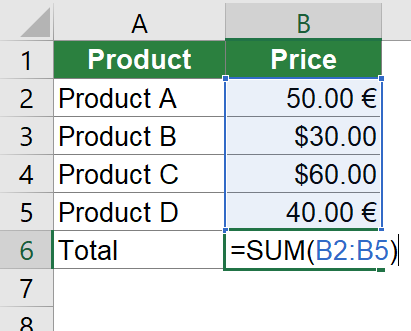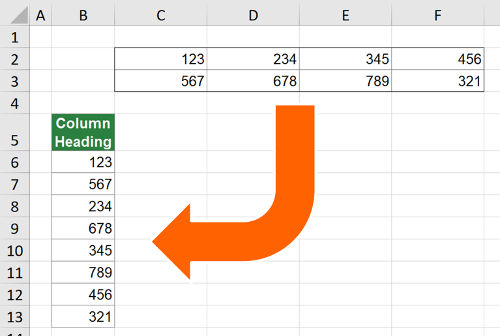#### Return Number Format Codes in Excel – 4 Ways to Get the Formatting Code from a Cell

Excel is a great software. It’s easy to use (at least the basic functions…) and very flexible. Unfortunately, coming with the flexibility, users tend to misuse the options and disobey certain basic rules. One thing I’ve seen multiple times is to transport important information in the formatting of a cell. It might be the background color, font color or strike-through. In this article, we’ll talk about something related: Return number format codes.Problem: Information is stored in the number format code of the Excel cell, for example the currency “USD” or “EUR”.

Let’s assume the following example. You receive a table containing prices with two columns. The first column contains the name of the item, for example “Product A”. The second column contains the respective prices. The problem is that instead of having one common currency, the currency information is only given in the number format code. Learn four methods in this article of how to return number format codes in Excel.

#### FIELDVALUE Formula in Excel: Insert Data of Companies and Countries (+Download)

Excel has – in it’s newest version – a quite useful new formula type. It’s called “linked data” and offers the functionality to automatically insert data from the internet to your table. This can be done with the FIELDVALUE formula and works in a first test quite well. Unfortunately, the available data types and options are limited so far. But let’s see how it works first.

#### Array Formulas in Excel: All You Need to Know

Array formulas are an advanced topic in Excel. Usually Excel users discover them when reaching the limits of – let’s call them – normal formulas, e.g. SUM, VLOOKUP, COUNT and so on. This article provides an introduction of array formulas in Excel.

A common task in Excel is to find out the number of different entries in a list. For example, you have a list of names and want to know, how many different people are listed as some people might be multiple times on the list. This article introduces 5 different methods of counting the number of unique records in a list, regarding two major differences.

1. You simply want to know the number of unique records. There is no other condition to be considered.
2. You want to know the number of different entries under one or more conditions.

FREQUENCY can classify data into bins. The formula in Excel is an array formula and just returns an array of values. In practice, it appears rather seldom. It is usually used within other formulas and not as a stand-alone formula. That said, let’s explore the purpose and usage of FREQUENCY.

#### Return Blank Cells Instead of Zeroes in Excel Formulas

If the return cell in an Excel formula is empty, Excel by default returns 0 instead. For example cell A1 is blank and linked to by another cell. But what if you want to show the exact return value – for empty cells as well as 0 as return values? This article introduces three different options for dealing with empty return values.

#### Convert Table to One Column in Excel: 4 Easy Methods to Copy All Columns underneath Each OtherCopy table to one column

Say you have an Excel table and want to copy all column underneath each other so that you only have one column. For example, you have a table 2 rows by 4 columns like in the screenshot on the right-hand side. You want to copy and paste this table to one column. You often need such transformation for inserting PivotTables or to create database formats. This article provides 4 simple methods to transform a 2-dimensional table into one column in Excel.